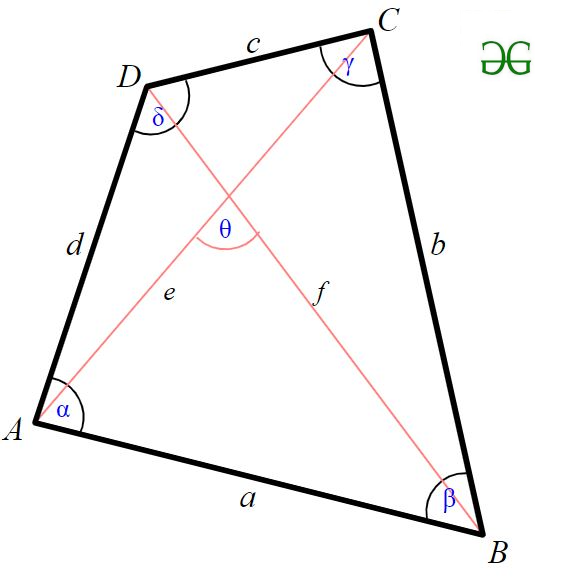Open in App
Not now

• Difficulty Level : Easy
• Last Updated : 22 Jun, 2022

Given four sides of quadrilateral a, b, c, d, find the maximum area of the quadrilateral possible from the given sides .
Examples:

Input : 1 2 1 2
Output : 2.00
It is optimal to construct a rectangle for maximum area .According to Bretschneider’s formula, the area of a general quadrilateral is given byHere a, b, c, d are the sides of a quadrilateral, s is the semiperimeter of a quadrilateral and angles are two opposite angles.
So, this formula is maximized only when opposite angles sum to pi(180) then we can use a simplified form of Bretschneider’s formula to get the (maximum) area K.This formula is called as Brahmagupta’s formula
Below is the implementation of given approach

## C++

 // CPP program to find maximum area of a  // quadrilateral #include  #include  using namespace std;    double maxArea(double a, double b,                 double c, double d) {     // Calculating the semi-perimeter      // of the given quadrilateral     double semiperimeter = (a + b + c + d) / 2;        // Applying Brahmagupta's formula to     // get maximum area of quadrilateral     return sqrt((semiperimeter - a) *                 (semiperimeter - b) *                  (semiperimeter - c) *                  (semiperimeter - d)); }    // Driver code int main() {     double a = 1, b = 2, c= 1, d = 2;    cout <

## C

 // CPP program to find maximum area of a  // quadrilateral #include  #include     double maxArea(double a, double b,                 double c, double d) {     // Calculating the semi-perimeter      // of the given quadrilateral     double semiperimeter = (a + b + c + d) / 2;        // Applying Brahmagupta's formula to     // get maximum area of quadrilateral     return sqrt((semiperimeter - a) *                 (semiperimeter - b) *                  (semiperimeter - c) *                  (semiperimeter - d)); }    // Driver code int main() {     double a = 1, b = 2, c= 1, d = 2;     printf("%.2f\n",maxArea(a, b, c, d));     return 0; }

## Java

 // Java program to find maximum area of a  // quadrilateral import java.io.*;   class GFG  {     static double maxArea(double a, double b,                            double c, double d)     {         // Calculating the semi-perimeter          // of the given quadrilateral         double semiperimeter = (a + b + c + d) / 2;               // Applying Brahmagupta's formula to         // get maximum area of quadrilateral         return Math.sqrt((semiperimeter - a) *                          (semiperimeter - b) *                           (semiperimeter - c) *                           (semiperimeter - d));     }           // Driver code     public static void main (String[] args)      {         double a = 1, b = 2, c= 1, d = 2;         System.out.println(maxArea(a, b, c, d));     } }   // This code is contributed by sunnysingh

## Python3

 # Python3 program to find maximum  # area of a quadrilateral import math   def maxArea (a , b , c , d ):       # Calculating the semi-perimeter     # of the given quadrilateral     semiperimeter = (a + b + c + d) / 2           # Applying Brahmagupta's formula to     # get maximum area of quadrilateral     return math.sqrt((semiperimeter - a) *                     (semiperimeter - b) *                     (semiperimeter - c) *                     (semiperimeter - d))   # Driver code a = 1 b = 2 c = 1 d = 2 print("%.2f"%maxArea(a, b, c, d))   # This code is contributed by "Sharad_Bhardwaj".

## C#

 // C# program to find maximum area of a  // quadrilateral using System;   class GFG {           static double maxArea(double a, double b,                           double c, double d)     {                   // Calculating the semi-perimeter          // of the given quadrilateral         double semiperimeter = (a + b + c + d) / 2;               // Applying Brahmagupta's formula to         // get maximum area of quadrilateral         return Math.Sqrt((semiperimeter - a) *                          (semiperimeter - b) *                           (semiperimeter - c) *                           (semiperimeter - d));     }           // Driver code     public static void Main ()      {         double a = 1, b = 2, c= 1, d = 2;                   Console.WriteLine(maxArea(a, b, c, d));     } }   // This code is contributed by vt_m.

## PHP

 

## Javascript

 

Output:

2.00`

Time Complexity: O(logn)
Auxiliary Space: O(1)

Please suggest if someone has a better solution which is more efficient in terms of space and time.